# Expected determinant of random symmetric matrix with different Gaussian distributions of the diagonal and non-diagonal elements

Consider a random matrix $$A \in \mathbb{R}^{N \times N}$$ where the elements are random gaussian variables. The mean and variance of the elements are different on the diagonal and the off-diagonal:

\begin{align} &\left=p &\text{and } & \text{var}(A_{ij})=\frac{1}{N}p(1-p) &\text{ if } \, i=j \\ &\left=p^2 &\text{and } &\text{var}(A_{ij})=\frac{1}{N} p^2(1-p^2) &\text{ if } \, i\neq j \end{align}

where $$p \in (0,1)$$ is some fixed paremeter. The matrix is symmetric $$A_{ij}=A_{ji}$$.

Now I would like to know

\begin{align} \mathbb{E}[\lim_{N\rightarrow \infty} |\det A|], \end{align}

i.e. the asymptotic behavior as $$N$$ becomes large. Since the variances are approaching zero for $$N \rightarrow \infty$$ I assume that the expectation of the absolute value of the determinant also approaches zero.

Is there a method to determine this behavior or does someone know how to do it?

Edit: I attached the following figure showing the result of Carlo Beenakker (lines)

$$\mathbb{E} [|\det A|] \sim N p^{N+1}(1-p)^{N-1}+p^{N}(1-p)^N$$

and results from simulations (crosses) for different $$p$$. They seem to fit very well.If I may ignore the fluctuations for large $$N$$, I can use that the average matrix $$\bar{A}=E(A)$$ has $$N-1$$ eigenvalues equal to $$p(1-p)$$ and one eigenvalue equal to $$p(1-p)+Np^2$$. The determinant then equals $${\rm det}\,\bar{A}=Np^{N+1}(1-p)^{N-1}+ p^N(1-p)^N.$$ Notice that it is positive definite. It decays exponentially $$\propto e^{-\alpha N}$$ with exponent $$\alpha=-\ln p-\ln(1-p)$$.

To assess the effect of the fluctuations I might consider the matrix $$\delta A=A-\bar{A}$$, where the matrix elements have zero average. The eigenvalues $$\lambda_n$$ of $$\delta A$$ are distributed according to the Wigner semicircle in the interval $$|\lambda|<2p(1-p)$$. That determinant can be at most $$2^{-N}$$ in absolute value, which also decays exponentially.

For $$p\lesssim 1/2$$ the Hermite polynomial formula (blue, absolute value of esg 's answer$$^*$$) is not far from $${\rm det}\,\bar{A}$$ (gold, above formula), see the plot for $$p=0.4$$ (at left) and for $$p=0.05$$ (at right):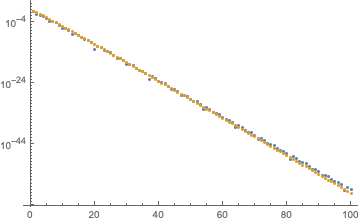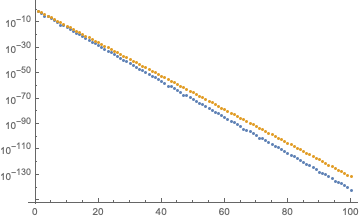For $$p>1/2$$ the Hermite polynomial formula decays less rapidly, but still exponentially: plots for $$p=0.7$$ (at left) and for $$p=0.95$$ (at right). The difference from the exponent $$\alpha$$ given above is close to a factor 1/2 for $$p$$ approaching 1 (compare with green curve $$\propto (1-p)^{N/2}$$).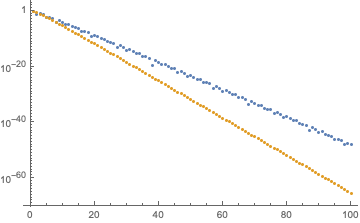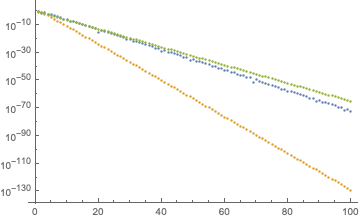In summary, based on this comparison I would conclude that the exponential decay of $$\mathbb{E}\,({\rm det}\,A)$$ crosses over from $$\propto p^N$$ for $$p$$ near 0 to $$\propto(1-p)^{N/2}$$ for $$p$$ near 1.

$$^*$$ I plotted the absolute value of the Hermite formula. It oscillates around zero with increasing $$p$$, here is for example a plot for $$N=100$$.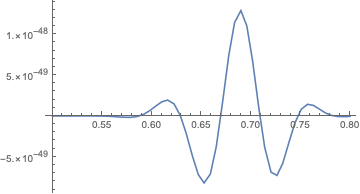• I compared the results for the average matrix without fluctuations with numerical results for the average absolute value of the determinant of A and they seem to fit very well. I will provide plots in a few days. Dec 24 '18 at 14:44

Here is a another approach. For convenience I write $$n$$ instead of $$N$$, and $$A_n$$ for $$A$$.

By definition

$$\det(A_n) = \sum_{\pi\in S_n} \operatorname{sign}(\pi) \prod_{i=1}^n a_{i,\pi(i)}$$

By assumption $$A_n$$ is symmetric and the $$a_{i,j}$$ are (for $$j\geq i$$) mutually independent random variables $$X_{i,j}$$ with $$\mathbb{E}(X_{i,i})=p=:a$$, $$\mathbb{E}(X_{i,j})=p^2=:c$$, and $$\mathbb{E}(X_{i,j}^2)=p^4 +\frac{p^2(1-p^2)}{n}=:b$$ for $$j\not =i$$.

Denote by $$C(\pi)$$ the set of cycles of $$\pi$$ and write $$\prod_{i=1}^n X_{i,\pi(i)}= \prod_{C \in C(\pi)} X_C$$, with $$X_C:=\prod_{i\in C} X_{i,\pi(i)}$$. The cycles are disjoint, thus the different $$X_C$$ are independent, further $$\mathbb{E}(X_C)=\left\{ \begin{array}{cr} a &\mbox{ if C is a fixed point}\\ b &\mbox{ if C is a 2-cycle (transposition)}\\ c^{|C|} &\mbox{ if the length |C| of C is \geq 3}\end{array}\right.$$

To use that, denote by $$Z_i(\pi), \,1\leq i\leq n$$ the no. of cycles of length $$i$$ of $$\pi$$ and by $$Z(\pi)=\sum_{i=1}^n Z_i(\pi)$$ denote the total no. of cycles of $$\pi$$, and recall that $$\operatorname{sign}(\pi)=(-1)^{n-Z(\pi)}$$ ( "a permutation $$\pi\in S_n$$ is even iff it's decrement $$n-Z(\pi)$$ is even"). We then have

$$\mathbb{E}\det(A_n)=\sum_{\pi\in S_n} (-1)^{n-Z(\pi)} a^{Z_1(\pi)} b^{Z_2(\pi)} c^{n-Z_1(\pi)-2Z_2(\pi)}$$

It is well known how to handle this type of sums. Standard generating function techniques (see e.g. section 5.2 in Stanley's EC 2) show that $$\sum_{\pi \in S_n} t^{Z(\pi)} t_1^{Z_1(\pi)}\ldots{t_n}^{Z_n(\pi)}=n! [z^n] e^{\sum_{i=1}^n t\,t_i\frac{z^i}{i}}$$ Using that and simple manipulations we find $$\mathbb{E}\det(A_n)=n!\,[z^n] (1+cz)\,e^{(a-c)z-(b-c^2)\frac{z^2}{2}}$$ The Hermite polynomials $$He_n(x)$$ are defined by $$He_n(x)=n![t^n] e^{xt-\frac{t^2}{2}}$$. Now note that with $$v:=b-c^2$$ (the variance of the off-diagonal matrix elements) and $$d:=a-c$$ (the difference of the expectations) you have $$\mathbb{E}\det(A_n)=\sqrt{v^n}\,He_n(\frac{d}{\sqrt{v}})+cn\sqrt{v^{n-1}}\,He_{n-1}(\frac{d}{\sqrt{v}})$$

The asymptotic properties of the Hermite polynomials have been intensively investigated, so you can probably find precise results for your concrete setting in the literature.

EDIT: Note that this doesn't answer your question for the expectation of the absolute value of the determinant (thanks to Carlo Beenakker for pointing this out). I'll still leave it here in case someone's interested in the expectation of the determinant.

• The OP asks for the expectation value of the absolute value of the determinant. Can your method be applied to that? I noticed that your answer oscillates around zero as a function of $p$ and I'm concerned that the averages of $|\det A|$ and $\det A$ might have different asymptotics. Dec 22 '18 at 9:38
• You're right, I failed to notice that. I don't think that the expectation of the absolute value of the determinant can be attacked by the same method. But note that $\mathbb{E}|\det(A)|\geq |\mathbb{E}\det(A)|$ so that the formula gives a lower bound. One might try to compute $\mathbb{E}(\det(A^2)$ in the same way, and use that for an upper bound (since here $\det(A^2)=|\det(A)|^2$, we have $\big(\mathbb{E}|\det(A)|\big)^2\leq \mathbb{E}\det(A^2)$).
– esg
Dec 22 '18 at 17:09

There is a similar question here : Expected value of determinant of simple infinite random matrix

I rewrite the heuristique from the random matrix theory: $$A=p^2 1 + (p-p^2)I_N+\sqrt{p^2(1-p^2)}\tilde{A}+\frac{1}{\sqrt{N}}D$$

where $$1$$ is the matrix with entries all equal to 1, $$D$$ a diagonal matrix with bounded variance. And $$A$$ is a matrix iid random entries with zero mean and variance $$1/N$$. Therefore its eigenvalues should be distributed similarly as the semicircul law. Because the first matrix is a rank one perturbation and $$\| \frac{1}{\sqrt{N}}D \|$$ is small, we could ignore them at first approximation. $$\frac{1}{N}\log(|\det(A)|)\sim \frac{1}{N}(\log(|\det(\frac{p-p^2}{\sqrt{p^2(1-p^2)}}I_N+\tilde{A}))|)+\log(\sqrt{p^2(1-p^2)})$$ We now use the semicircul law and we should get $$\frac{1}{N}(\log(|\det(\Lambda I_N+\tilde{A})|))=\frac{1}{N}\sum_{\lambda \in \sigma (\tilde{A})} \log (|\Lambda+\lambda|) \sim \int_{-2}^{2} \log(|\Lambda+\lambda|) \rho(\lambda) d\lambda$$ where $$\Lambda=\frac{p-p^2}{\sqrt{p^2(1-p^2)}}$$ and $$\rho(\lambda ) = \frac{\sqrt{4-\lambda^2}}{2\pi}$$ is the semicircul law. So finally we should obtain $$\frac{1}{N}\log(|\det(A)|)\sim \log(\sqrt{p^2(1-p^2)})+\int_{-2}^{2} \log(|\Lambda+\lambda|) \rho(\lambda) d\lambda$$